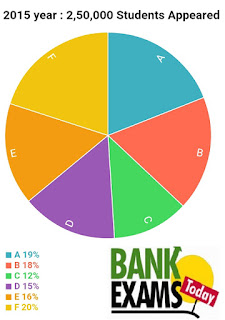New Students Offer - Use Code HELLO

# Expected Data Interpretation Set for IBPS PO - Part 6

### Study the following pie-charts carefully and answer the following questions

The following pie-chart show the number of students appearing GATE Examination From different states .1. Number of students appearing from B state in 2016 was , What per cent of that from F state F in 2015 ?

1.94
2.95
3.96
4.97
5.98

2. The total number of students appearing from B & C together in 2015 is approximately equal to that from which of the following pairs of states in 2016 ?

1.A&F
2.C&D
3.A&C
4.D&F
5.E&B

3.If there were 30000 students appearing in the examination from Hyderabad in 2016,and 35000 students appearing from Chennai in 2016, find the percentage of students students not from the Hyderabad and Chennai in 2016.

1.70.87
2.79.56
3.76.09
4.72.91
5.76.85

4.What is the per cent students appearing from state A,C and F in 2015 , same states in 2016 ?

1.98.37
2.96.89
3.92.78
4.91.56
5.89.78

5. If number of students appearing examination in 2014 is 20% more than students appearing in 2015 , Find the number of students appearing examination from state B in 2014 ?

1.56%
2.67%
3.76%
4.Data Insufficient
5.None of the above

1.3

No.of students appearing from B state in 2016 = 20*240000/100 = 48000

No.of students appearing from F state in 2015 = 20*250000/100 = 50000

Percentage = 48000*100/50000 = 96%

2.3

Students appearing from B and C in 2015 = (18+12)*250000/100 = 75000

Go through options verification check one by one

You will find option C is approximately equal to 75000

3.4

Students from Hyderabad and Chennai in 2016= 30000+35000=650000

Total number of students in 2016 = 240000

Students not from Chennai and Hyderabad = 240000-65000= 175000

Percentage = 175000*100/240000 = 72.91

4.1

Students appearing from A,C,and F in 2016 = (13+18+23)*240000/100= 129600

Students appearing from A,C and F in 2015 = (19+12+20)*250000/100 = 127500

Percentage = 127500/129600 * 100 = 98.37

5.4

Students appearing examination is 20% more than the students appearing examination in 2015 = 300000

But F state details in 2014 not given